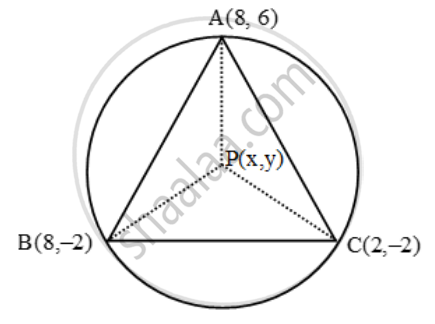# Find the coordinates of the circumcentre of the triangle whose vertices are (8, 6), (8, – 2) and (2, – 2). Also, find its circum radius - Mathematics

Sum

Find the coordinates of the circumcentre of the triangle whose vertices are (8, 6), (8, – 2) and (2, – 2). Also, find its circum radius

#### Solution

Recall that the circumcentre of a triangle is equidistant from the vertices of a triangle. Let A (8, 6), B(8, –2) and C(2, – 2) be the vertices of the given triangle and let P (x, y) be the circumcentre of this triangle. Then,

PA = PB = PC ⇒ PA^2 = PB^2 = PC^2Now, PA2 = PB2

⇒ (x – 8)^2 + (y – 6)^2 = (x – 8)2 + (y + 2)^2

⇒ x^2 + y^2 – 16x – 12y + 100 = x^2 + y^2 – 16x + 4y + 68

⇒ 16y = 32 ⇒ y = 2

and, PB2 = PC2

⇒ (x – 8)^2 + (y + 2)^2 = (x – 2)^2 + (y + 2)^2

⇒ x^2 + y^2 – 16x + 4y + 68 = x^2 + y^2 – 4x + 4y + 8

⇒ 12x = 60 ⇒ x = 5

So, the coordinates of the circumcentre P are (5, 2).

Also, Circum-radius = PA = PB = PC

=sqrt((5-8)^2+(2-6)^2)=\sqrt{9+16}=5

Concept: Distance Formula
Is there an error in this question or solution?

Share